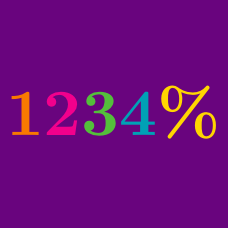Everyday Math

# Percentages In Calculation

Is this statement true or false?

Mike can find 3% of any number by finding 1% of the number and multiplying that value by 3.

True or False?

The percentage wage increase of $20 per hour to$24 per hour is greater than the percentage wage increase of $15 per hour to$18 per hour.

If a positive number is doubled, its value increases by which percent?

A rectangle's width is 40% of its length. If the perimeter of the rectangle is 56 units, what is the area of the rectangle?

The number of people owning memberships at a gym increased by 20% to 60. Did the gym have 40 or 50 members before the increase?

×